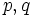# Order has only two prime factors implies solvable

This article gives the statement and possibly, proof, of an implication relation between two group properties. That is, it states that every group satisfying the first group property (i.e., group whose order has at most two prime factors) must also satisfy the second group property (i.e., solvable group)
View all group property implications | View all group property non-implications
Get more facts about group whose order has at most two prime factors|Get more facts about solvable group

## Statement

The Burnside's p^aq^b theorem states that a group whose order has at most two prime factors (viz., a group whose order is of the form$p^aq^b$ where$p,q$ are primes and$a,b$ are nonnegative integers), must be solvable.

This includes the case of the trivial group as well as of a group of prime power order, though much stronger statements can be made in these cases.

## Facts used

The table below lists key facts used directly and explicitly in the proof. Fact numbers as used in the table may be referenced in the proof. This table need not list facts used indirectly, i.e., facts that are used to prove these facts, and it need not list facts used implicitly through assumptions embedded in the choice of terminology and language.
Fact no. Statement Steps in the proof where it is used Qualitative description of how it is used in the proof What does it rely on? Other applications
1 Sylow subgroups exist Step (1) of first part of proof Constructs a$p$-Sylow subgroup, which is then shown to be contained in the centralizer of a non-identity element. Basic group theory, group actions click here
2 Prime power order implies not centerless Step (1) of first part of proof Find a central element in a$p$-Sylow subgroup, which must therefore have a fairly big centralizer in the whole group. Basic group theory, class equation of a group click here
3 Simple abelian implies cyclic of prime order Step (2) of first part of proof Takes care of the case of nontrivial center Basic group theory click here
4 Conjugacy class of prime power size implies not simple Step (3) of first part of proof This fact itself is the hardest part of the proof, though its application to the proof is straightforward. Character theory; hardest part of proof click here
5 Lagrange's theorem Step (1) of first part of proof, inductive step of second part of proof Reducing from a group to a normal subgroup Basic group theory click here
6 Order of quotient group divides order of group Inductive step of second part of proof Reducing from a group to a quotient group Basic group theory click here
7 Solvability is extension-closed Inductive step of second part of proof Putting together solvability of normal subgroup and quotient group to get solvability of whole group Basic definition of solvability click here

## Proof

This proof uses a tabular format for presentation. Provide feedback on tabular proof formats in a survey (opens in new window/tab) | Learn more about tabular proof formats|View all pages on facts with proofs in tabular format
This proof uses the principle of mathematical induction in a nontrivial way (i.e., it would be hard to write the proof clearly without explicitly using induction).

### First part of proof: a nontrivial group whose order has only two prime factors is not simple unless it is cyclic of prime order

Given: A nontrivial group$G$ whose order is$p^aq^b$ for primes$p,q$ and nonnegative integers$a,b$.

To prove:$G$ is either cyclic of prime order or it is not simple.

Proof: Without loss of generality, assume that$a > 0$ (if both$a = b = 0$,$G$ is trivial and there is nothing to prove).

Step no. Assertion/construction Facts used Given data used Previous steps used Explanation
1 Either the center of$G$ is nontrivial, or$G$ has a conjugacy class of prime power order Facts (1), (2), (5)$|G| = p^aq^b$ [SHOW MORE]
2 If the center of$G$ is nontrivial, then$G$ is either cyclic of prime order or not simple. Fact (3) [SHOW MORE]
3 If$G$ has a conjugacy class of prime power order, then$G$ is not simple. Fact (4) Fact-direct.
4$G$ is either cyclic of prime order or not simple. Steps (1),(2),(3) Step-combination-direct.

### Second part of proof: using induction

We prove the statement by a strong form of induction on the order. Specifically, the statement we are trying to prove is:

Statement to be proved by induction on$n$: If$n$ is of the form$p^aq^b$ where$p,q$ are primes and$a,b$ are nonnegative integers, then any group of order$n$ is solvable. Note that this includes the values of$n$ that are powers of only a single prime and it also includes$n = 1$, because$a,b$ are allowed to be zero.

Our strong form of induction uses the truth of the statement for all smaller orders. In other words:

Inductive hypothesis: The statement to be proved by induction is true for all$m < n$. In particular, it is true for all proper divisors of$n$.

Inductive goal: If$n = p^aq^b$ with$p,q$ primes and$a,b$ nonnegative integers, and$G$ is a group of order$n$, then$G$ is solvable.

Proof: We make two cases:

1. Case that$G$ is simple: In this case, the first part of proof yields that$G$ is cyclic of prime order, hence abelian, and hence solvable.
2. Case that$G$ is not simple: In this case, there is a proper nontrivial normal subgroup$N$ of$G$. By facts (5) and (6), the orders of$N$ and$G/N$ both divide the order of$G$, and hence both have at most two prime factors. Hence, the induction hypothesis applies to both (since$N$ is proper and nontrivial), and we obtain that$N$ and$G/N$ are both solvable. Thus, by fact (7),$G$ is solvable.